# Math 201 - All Lessons

A collection of elementary math projects that demonstrate the application of coding to solve math problems and model math concepts.

• INTERMEDIATE

1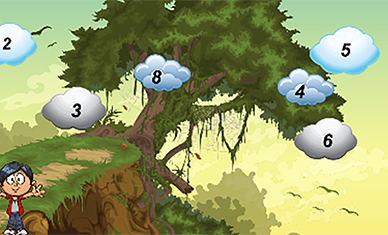Math Quiz
Create a quiz that demonstrates your knowledge of math.

2Coordinate Plane
Create a project that demonstrates your knowledge of coordinate planes.

3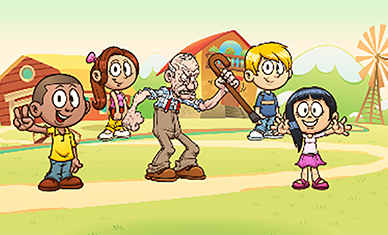Average Age
Create a project that demonstrates your knowledge of calculating averages.

4Positive and Negative Values
Create a project that demonstrates your knowledge of positive and negative values.

5Double Negatives
Create a project that demonstrates your knowledge of double negatives.

6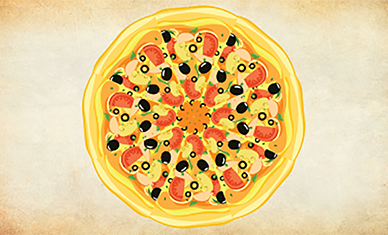Percents and Money
Create a project that demonstrates your knowledge of percents and money.

7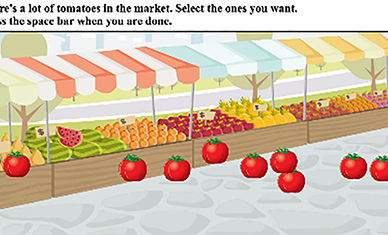Market Price
Create a project that demonstrates your knowledge of percentages and calculating the price of items.

8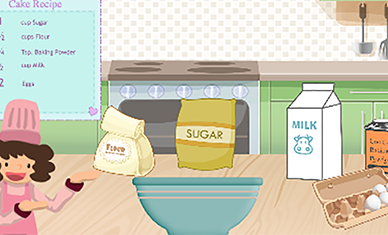Create a project that demonstrates your knowledge of math to follow recipes.

9My Shop
Create a project that demonstrates your knowledge of math.

10Probability Magic
Create a project that demonstrates your knowledge of probability.

11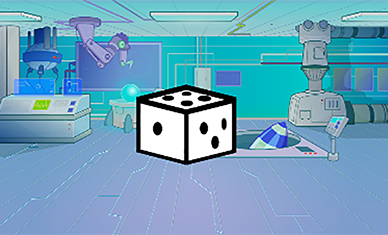Dice Rolls
Create a project that demonstrates your knowledge of probability.